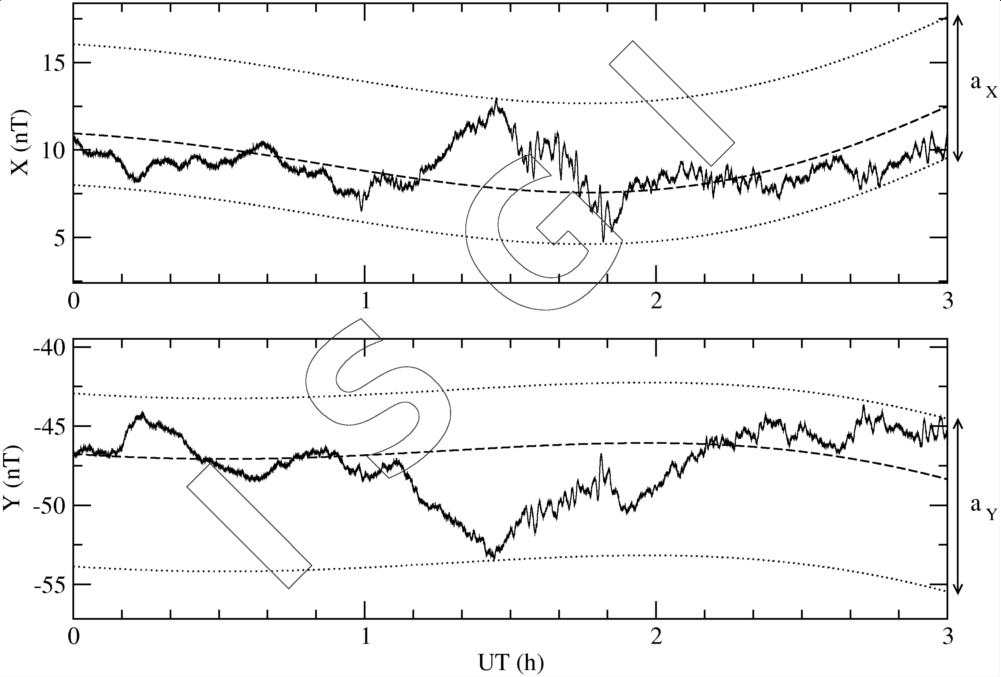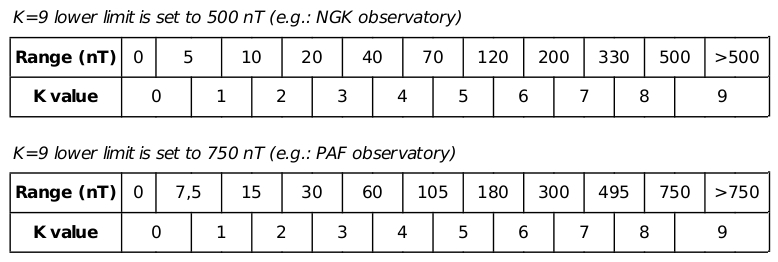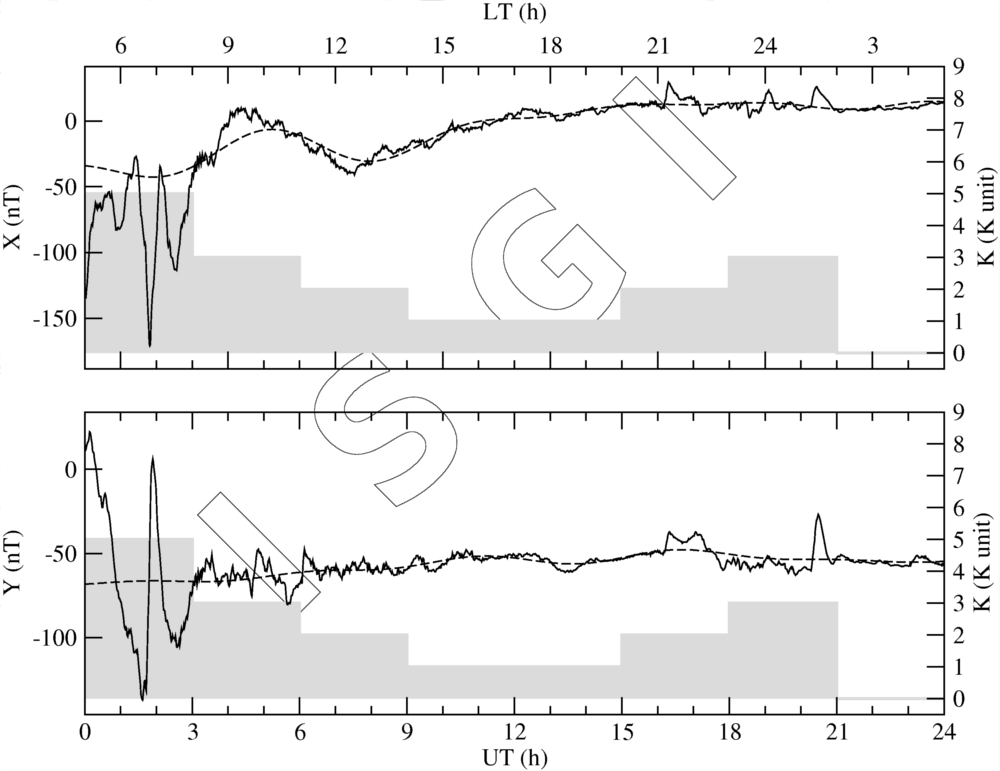Service International des Indices Géomagnétiques
International Service of Geomagnetic Indices

# What are K indices?

Definition: An individual K index is an integer in the range 0 to 9 corresponding to a class that contains, after eliminating the regular daily variation (SR), the largest range of geomagnetic disturbances (aX and aY) in the two horizontal components (X and Y) during a 3-hour UT interval.
The limits of these classes at a particular observatory are defined with the intent of producing a geomagnetic disturbance characterisation that does not depend significantly on the location of a sub-auroral, mid- or low- latitude observatory.

 Example: SR and ranges (aX and aY) determination [00-03 UT three hour interval on 2010, April 11th at the PAF observatory] For both X and Y components, the dashed line represents the estimated SR variation, and the upper and lower dotted lines correspond to the SR curve shifted respectively up to the maximum and down to the minimum of the observed magnetic field deviation from the SR baseline. aX and aY (respectively equal to 7.5 and 9.8 nT) correspond to the three hour ranges from which the K index is derived (K = 1 in this example).Limits of class: The limits of classes at a particular observatory are defined with the intent of producing a geomagnetic disturbance characterisation that does not depend significantly on the location of a sub-auroral, mid- or low- latitude observatory.
The limits may vary from one observatory to another since they depend on the corrected geomagnetic latitude.

 The mandatory value is the so-called "K=9 lower limit" (also nammed L9 value) which allows to determine, for each magnetic observatory, the conversion table between classes of ranges and K indices. Example: The NGK table is the reference defined by Mayaud. Then to obtain PAF table, NGK's classes of range limits are multiply by the (L9PAF / L9NGK)= (750 / 500) factor.ISGI-headquarters are in charge of the computation of L9 value for each magnetic observatory.

Computation of K indices: The original definition of K indices (Bartels et al., 1939) requires hand scaling on analogue magnetograms. The question of the derivation of geomagnetic indices from digital data arose at the end of the seventies.

 Different algorithms enabling computer derivation of K indices were then developed and carefully assessed in the frame of an international comparison organised by the IAGA Working Group “Geomagnetic indices” (Coles & Menvielle, 1991; Menvielle, 1991; Menvielle et al., 1995). Basically, these algorithms estimate the SR variation from the magnetograms. The geomagnetic disturbances, from which the K indices are computed, are derived as the difference between the observed variations and the computer estimated SR ones. Example: SR and K determination by the FMI algorithm (Sucksdorff et al., 1991) for a moderately disturbed day (2010, April 12th; Am = 30 nT) at the PAF observatory.